• 2021-04-21 16:33:34

plot 是绘制二维图形的最基本函数，它是针对向量或矩阵的列来绘制曲线的。也就是说，使用plot 函数之前，必须首先定义好曲线上每一点的x 及y 坐标。

1. plot(x)

当x 为一向量时，以x 元素的值为纵坐标，x 的序号为横坐标值绘制曲线。当x 为一实矩阵时，则以其序号为横坐标，按列绘制每列元素值相对于其序号的曲线。

2. plot(x，y)

以x 元素为横坐标值，y 元素为纵坐标值绘制曲线

3. plot(x，y1，x，y2，…。)

plot(x，y1，x，y2，…) 以公共的x 元素为横坐标值，以y1，y2，… 元素为纵坐标值绘。

制多条曲线

4. 绘图参数表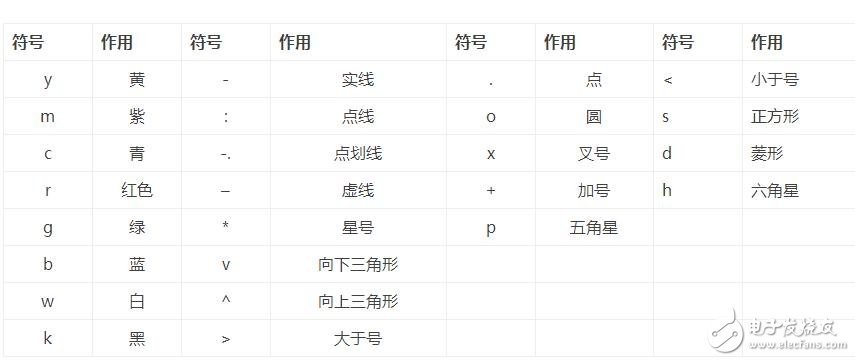例如

plot(x，y1，‘r+-’，x，y2，‘k*：’)1

函数含义

grid on (/off) 给当前图形标记添加(取消)网络

xlable(‘string’) 标记横坐标

ylabel(‘string’) 标记纵坐标

TItle(‘string’) 给图形添加标题

text(x，y，’string’) 在图形的任意位置增加说明性文本信息

gtext(‘string’) 利用鼠标添加说明性文本信息

axis(［xmin xmax ymin ymax］) 设置坐标轴的最小最大值

更多相关内容
• plot3函数与plot函数的使用方式相近。plot函数具体可参见：https://blog.csdn.net/Mrweng1996/article/details/104338453 1、plot3函数的基本用法： plot3(x, y, z)：其中，x、y、z组成一组曲线的坐标。 代码示例...

plot3函数与plot函数的使用方式相近。plot函数具体可参见：https://blog.csdn.net/Mrweng1996/article/details/104338453

### 1、plot3函数的基本用法：

plot3(x, y, z)：其中，x、y、z组成一组曲线的坐标。

代码示例：

t = linspace(0,10*pi,200);
x = sin(t) + t.*cos(t);
y = cos(t) - t.*sin(t);
z = t;
subplot(1,2,1);
plot3(x, y, z);
grid on
subplot(1, 2, 2);
plot3(x(1:4:200), y(1:4:200), z(1:4:200));
grid on

运行结果：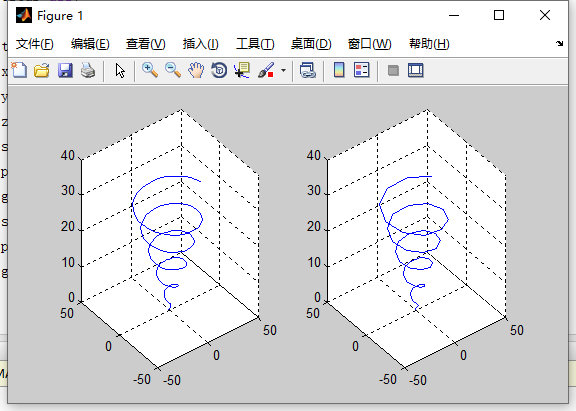### 2、 plot3函数参数的变化形式

plot3(x, y, z)

参数x，y，z为同型矩阵。

参数x，y，z中有向量，有矩阵。

代码示例：

clc;
clear all;

t = 0:0.01:2*pi;
t = t';
x = [t,t,t,t,t];
y = [sin(t),sin(t)+1,sin(t)+2,sin(t)+3,sin(t)+4];
z = [t,t,t,t,t];
plot3(x,y,z);

运行结果：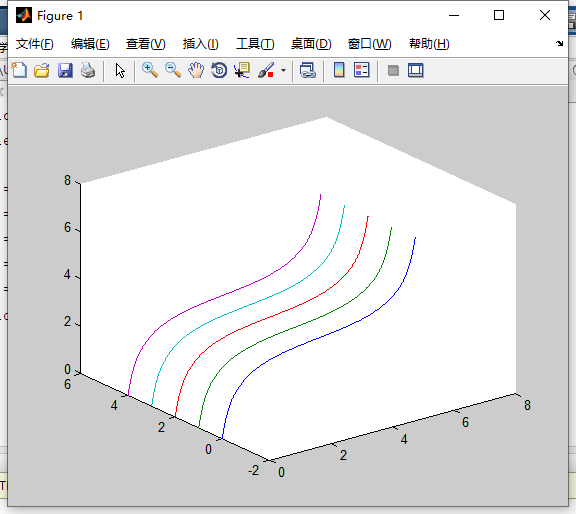### 3、含有多组输入参数的plot3函数

plot3(x1, y1, z1, x2, y2, z2 ..., xn, yn, zn) ：每一组x、y、z组成一组数据点的坐标，绘制一条曲线。

clc;
clear all;

t1 = 0:0.01:1.5*pi;
t2 = 0:0.01:2*pi;
t3 = 0:0.01:3*pi;
plot3(t1, sin(t1), t1, t2, sin(t2)+1, t2,t3, sin(t3)+2, t3)
grid on

运行结果：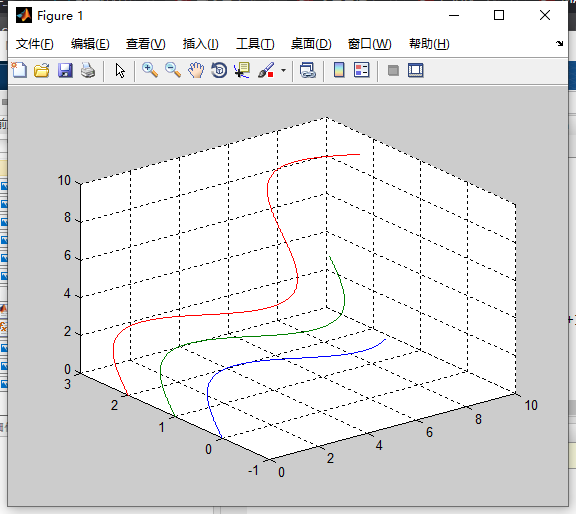### 4、含有选项的plot3函数

plot(x, y, z, 选项)：选项适用于指定曲线的线型、颜色和数据点标记。

代码示例：

clc;
clear all;

t = 0:pi/50:6*pi;
x = cos(t);
y = sin(t);
z = 2*t;
plot3(x, y, z, 'p');
xlabel('X')
ylabel('Y')
zlabel('Z')
grid on


运行结果：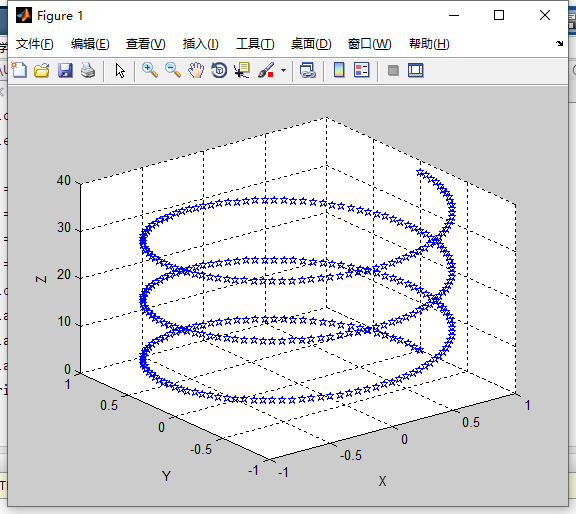展开全文• 1、plot3函数 ( 1 ) plot3函数的基本用法 plot3(x，y，z) 其中，参数x、y、z组成一组曲线的坐标。 例1：绘制一条空间折线。 x = [0.2 1.8 2.5]; y = [1.3 2.8 1.1]; z = [0.4 1.2 1.6]; plot3(x,y,z) title('三维...1、plot3函数
( 1 ) plot3函数的基本用法
plot3(x，y，z)

其中，参数x、y、z组成一组曲线的坐标。

例1：绘制一条空间折线。

x = [0.2 1.8 2.5];
y = [1.3 2.8 1.1];
z = [0.4 1.2 1.6];
plot3(x,y,z)
title('三维曲线','color','r')
grid on
axis([0,3,1,3,0,2])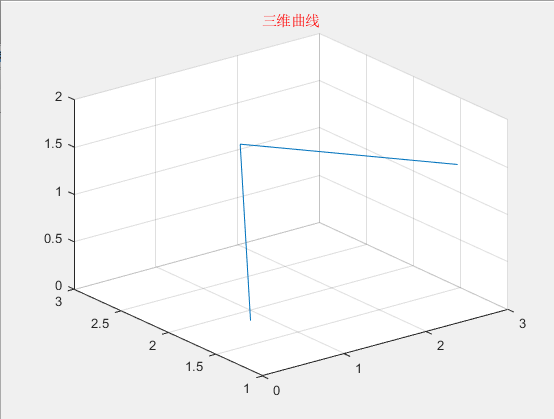t = linspace(0,14*pi,200);
x = sin(t)+t.*cos(t);
y = cos(t)-t.*sin(t);
z = t;

subplot(1,2,1)
plot3(x,y,z)    %有200个数据点，曲线较光滑
grid on

subplot(1,2,2)
plot3(x(1:3:200),y(1:3:200),z(1:3:200))   %有67个数据点，曲线较粗糙
grid on

% subplot(1,2,2)
% t1 = linspace(0,14*pi,80);
% x1 = sin(t1)+t1.*cos(t1);
% y1 = cos(t1)-t1.*sin(t1);
% z1 = t1;
% plot3(x1,y1,z1)
% grid on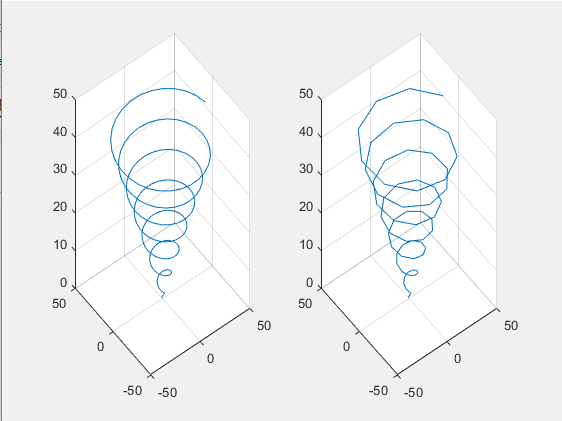( 2 ) plot3函数参数的变化形式
plot3(x, y,z)

①参数x、y、z是同型矩阵：则以XYZ对应列元素绘制曲线，曲线条数等于短阵列数。
②参数x、y、z中有向量，也有矩阵：向量的长度应与矩阵相符，行向量的长度与矩阵的列数相同，列向量的长度与矩阵的行数相同。

例3：在空间不同位置绘制5条正弦曲线。

方法1  列向量
t = linspace(0,2*pi,100);
t = t';
x = [t t t t t];
y = [sin(t) sin(t)+1 sin(t)+2 sin(t)+3 sin(t)+4];
z = [t t t t t];
plot3(x,y,z)
grid on
legend('sin(t)','sin(t)+1','sin(t)+2','sin(t)+3','sin(t)+4','location','north')

方法2  行向量
t = linspace(0,2*pi,100);
x = t;
y = [sin(t);sin(t)+1;sin(t)+2;sin(t)+3;sin(t)+4];
z = t;
plot3(x,y,z)
grid on
legend('sin(t)','sin(t)+1','sin(t)+2','sin(t)+3','sin(t)+4','location','north')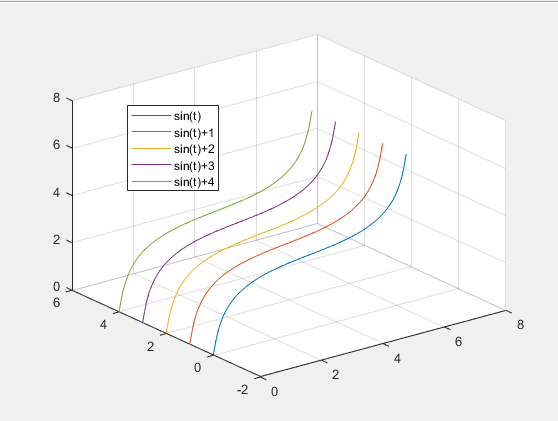(3)含多组输入参数的plot3函数
plot3(x1, y1, z1,x2,y2,z2，…, xn, yn, zn)

每一组x、y、z向量构成一组数据点的坐标，绘制一条曲线。

例4：绘制三条不同长度的正弦曲线。

t1 = 0:0.01:1*pi;
t2 = 0:0.01:2*pi;
t3 = 0:0.01:3*pi;
plot3(t1,sin(t1),t1,t2,sin(t2)+1,t2,t3,sin(t3)+2,t3)
legend('sin(t1)','sin(t2)+1','sin(t3)+2','location','north')
grid on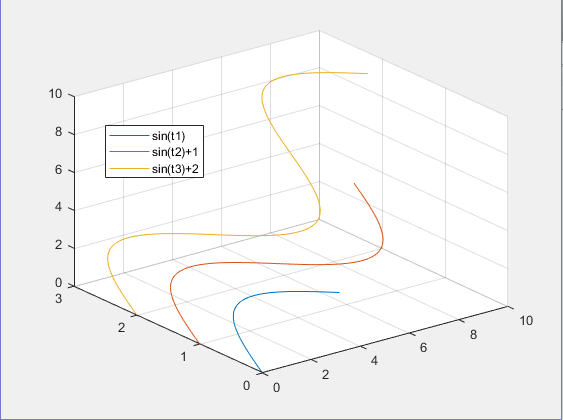(4)含选项的plot3函数
plot3(x, y,z,选项)

选项用于指定曲线的线型、颜色和数据点标记。t = 0:pi/50:6*pi;
x = cos(t);
y = sin(t);
z = 2*t;
plot3(x,y,z,'p')   %p以五角星输出
xlabel('X')
ylabel('Y')
zlabel('Z')
grid on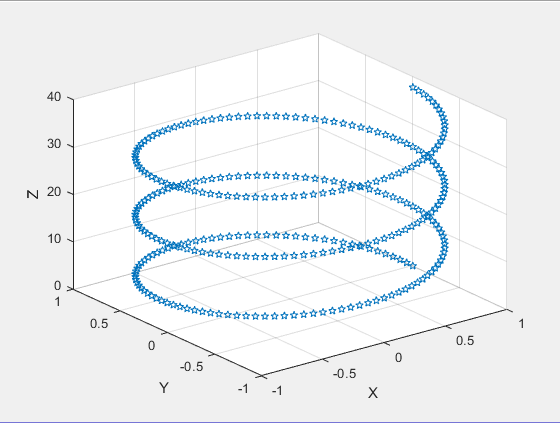2、fplot3函数
fplot3(funx, funy, funz, tlims)
其中, funx、funy、funz代表定义曲线x、y、z坐标的函数，通常采用函数句柄的形式。tlims为参数函数自变量的取值范围，用二元向量[tmin，tmax]描述﹐默认为[-5，5]。xt = @(t)exp(-t/10).*sin(5*t);
yt = @(t)exp(-t/10).*cos(5*t);
zt = @(t)t;
fplot3(xt,yt,zt,[-12,12],'b')
xlabel('X')
ylabel('Y')
zlabel('Z')
title('墨西哥冒顶曲线','fontsize',15,'color','r')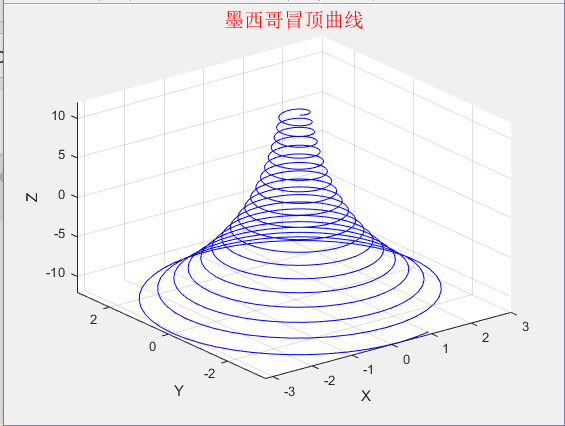展开全文• ## MATLAB中plot函数的用法

万次阅读 多人点赞 2018-04-23 15:47:04
使用plot绘制二维图像本文转自http://blog.sina.com.cn/s/blog_d8f783c90102woqb.html以及https://blog.csdn.net/alvern_zhang/article/details/51153058MATLABplot函数常常被用于绘制各种二维图像，其用法也是...

## 使用plot绘制二维图像

本文转自http://blog.sina.com.cn/s/blog_d8f783c90102woqb.html

以及https://blog.csdn.net/alvern_zhang/article/details/51153058

MATLABplot函数常常被用于绘制各种二维图像，用法也是多种多样，本文仅介绍plot函数的基本用法——使用plot函数绘制二维点图和线图。plot函数的一般调用形式如下：

plot(X, Y, LineSpec)

其中X由所有输入点坐标的x值组成，Y是由与X中包含的x对应的y所组成的向量。LineSpec是用户指定的绘图样式，主要选项如下：

 Specifier Line Style（线型） --- 实线（默认样式）虚线（短划线） : 点线 -. 点划线

 Specifier Marker（坐标点样式） o 圆 + 加号 * 星号 . 点 x 十字 s 正方形 d 菱形 ^ 上指向三角形 v 下指向三角形 > 右指向三角形 < 左指向三角形 p 五角星 h 六角形

 Specifier Color y 黄色 m 品红 c 蓝绿色 r 红色 g 绿色 b 蓝色 w 白色 k 黑色

例如：’--or’表示坐标点为圆圈标志，且线型为红色短划线的绘图样式。

### 注意

在同时绘制多条曲线时，如果没有指定曲线属性，plot按顺序循环使用当前坐标系中ColorOrder和LineStyleOrder两个属性。

默认情况，MATLAB在每次调用plot函数时将ColorOrder和LineStyleOrder自动重置为DefaultAxesColorOrder和DefaultAxesLineStyleOrder。Default**属性我们可以自定义，有效期至MATLAB关闭，Matlab下次启动时将Default**属性重置为厂家设置(Factory)

set(0,’DefaultAxesColorOrder’,’r|g|b|k’,…’DefaultAxesLineStyleOrder’,’-|-.|–|:’)

使用hold all命令可以阻止调用plot函数时自动重置ColorOrder和LineStyleOrder属性，而是循环使用。注意hold on只是使多次绘制的图形叠加（相当于NextPlot），但不能阻止属性重置。

另外我们可以通过下面四个属性设置标识符的颜色和大小

LineWidth——指定线宽

MarkerEdgeColor——指定标识符的边缘颜色

MarkerFaceColor——指定标识符填充颜色

MarkerSize——指定标识符的大小

注意上面四个属性是针对当前坐标系中所有曲线的

### 实例

X=1:10;
% 两个都是数组，必须具有相同的尺寸
X1=[X;X;X]’;%10×3
Y1=rand(10,3)+1;%10×3% 其中一个为向量，另一个为数组，自动匹配尺寸相等方向
X2=1:0.1:10;%1×91
Y2=[sin(X2);cos(X2)]’;%91×2% 其中一个是标量，另一为矢量，绘制垂直坐标轴的离散点
X3=1:10;
Y3=-0.5;
fh=figure(‘numbertitle’,’off’,’name’,’PLOT Usability Demo’);%创建figure对象
ah=axes;%创建axes对象
h=plot(…%返回所有曲线句柄
ah,…%指定坐标系，可以省略，此时默认gca
X1,Y1,…%坐标数据
‘-.^’,…%曲线属性，可以省略或部分省略，此时自动选择
X2,Y2,…
‘m-‘,…
X3,Y3,…
‘o’,…%注意此组数据设置线型和颜色无效，因为默认绘制离散点
‘LineWidth’,2,…%线宽
‘MarkerEdgeColor’,’k’,…%标识符边缘颜色
‘MarkerFaceColor’,’r’,…%标识符填充颜色
‘MarkerSize’,8)%标识符大小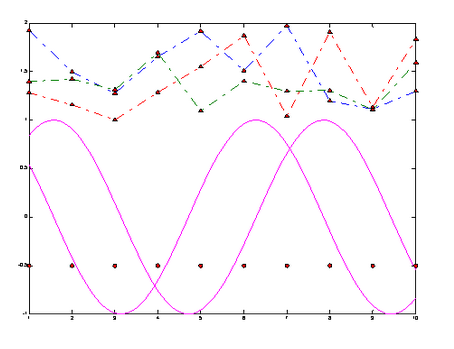举例如下：

定义x02pi之间的一组向量，且x向量中相邻两个值之间的增量为pi/100。定义y向量中的值为x向量的sine值。

x = 0:pi/100:2*pi;

y = sin(x);

figure 打开新的绘画窗口，可省略该句

plot(x,y) %采用默认样式，绘制实线

x = 0:pi/20:2*pi;

y = sin(x);

plot(x,y,'--or') %坐标点为圆圈标志，且线型为红色短划线的绘图样式

注：可以根据上述LineSpec表格自由组合线型样式。

在不关闭绘图窗口的前提下，还可以为图像添加标题，x轴和y轴标签，例如：

xlabel('x')

ylabel('sin(x)')

title('Plot of the Sine Function')

想要在现有的图片上添加另外一条线，可以使用hold语句，例如：

x = 0:pi/100:2*pi;

y = sin(x);

plot(x,y)

hold on

y2 = cos(x);

plot(x,y2,'r:')

legend('sin','cos') %为图片添加图例## 使用plot3绘制三维图像

MATLAB中有许多函数可以用来绘制三维图像，在此仅对plot3函数进行简要介绍，有兴趣的同学可以自行学习。plot3函数的调用形式如下：

plot3(X, Y, Z,LineSpec)

例如：

z=0:pi/50:10*pi;

x=sin(z);

y=cos(z);

plot3(x,y,z)

展开全文• 1、 plot函数的基本用法 plot(x, y)：其中，x和y分别存储x坐标与y坐标。 代码示例： clc; clear all; x = [1,3,4,6]; %x数据 y = [2,3,4,5]; %y数据 plot(x,y); 运行结果： 2、最简单的plot函数的调用格式 plot(x...
• 三维绘图基本流程 三维绘图的基本流程如下： （1）数据准备； （2）图形窗口和绘图区选择；...与这条命令类似，MATLAB也提供了一个绘制三维折线或曲线的基本命令plot3。 该命令的格式如下。 plot2(x1,y1,z1,opti
• 文章目录0 前言1 plot3函数1.1 plot3函数的基本用法1.2 plot3（x，y，z）函数参数的变化形式1.3 含多组输入参数的plot3函数1.4 含选项的plot3函数2 fplot3函数2.1 fplot3函数的基本用法2.2 练习3 结语 0 前言 本文是...
• matlab_plot函数用法 matlab_plot函数用法 matlab_plot函数用法 matlab_plot函数用法 matlab_plot函数用法 matlab_plot函数用法
• ## MATLAB：plot函数详解

万次阅读 多人点赞 2021-08-08 20:50:29
本文介绍了MATLABplot函数的用法，方便日后适用
• ## matlab中plot函数用法

万次阅读 多人点赞 2019-03-06 14:41:15
1.简单的2维直线图 : plot(x,y) 同一坐标显示n条线：plot(x,y1,x,y2，…) x = 0:pi/10:2*pi; y = sin(x); figure; hold on; plot(x,y) 2.plot(X):X是矩阵，表示矩阵的每一行都画一条线，将显示n条线。 X=rand(3,3)...
• ## matlabplot绘图函数官方详解

万次阅读 多人点赞 2020-06-28 22:47:24
二维线图函数 语法 plot(X,Y) plot(X,Y,LineSpec) plot(X1,Y1,...,Xn,Yn) plot(X1,Y1,LineSpec1,...,Xn,Yn,LineSpecn) plot(Y) plot(Y,LineSpec) plot(___,Name,Value) plot(ax,___) h = plot(___) ...
• ## matlabplot函数详解

万次阅读 多人点赞 2018-04-28 17:04:08
matlab中，plot函数用来绘制二维图像。1.plot默认格式 plot(x,y)这种格式中，若x,y是向量，则它们必须具有相同的长度。函数将以x为横轴，绘制y。 若x,y都是矩阵，则它们必须具有相同的尺寸，plot函数将针对x的各...
• 本代码主要利用MATLAB工具实现MATLAB——semilogx函数和plot函数指令对比，简单明了，易于理解
• plot（x，y，’color’，’颜色’） 二．形状 1. 连续点 - 实线 –（这是两个线- -） 虚线 ： 虚线 -. 点线 2. 离散点 离散点 把离散点连起来 + -+ o（注意是英文o，不是数字...
• 这是如何从 MATLAB:registered: 中的函数创建线图的示例。 阅读 MATLAB 文档中的“fimplicit”函数。 此功能在 R2016b 或更新版本中可用。 有关更多示例，请转到 MATLAB 绘图库 - ...
• plot(t,sin(t),'--mo','LineWidth',2,'MarkerEdgeColor','k','MarkerFaceColor',[.49 1 .63],'MarkerSize',12); %'--mo',这里是设置线型（--）和颜色（m），标记点类型(o，没有变默认为连续），后面一次是线的宽度...
• (1)当x是实向量时，则绘制出以该向量元素的下标(即向量的长度，可用MATLAB函数length求得)为横坐标，以该向量元素的值为纵坐标的一条连续曲线。 >> x=[10,20,30,40,50]; >> plot(x) (2)当x是实矩阵时...
• 文章目录前言一、plot()函数二、代码运行结果总结 前言 此为本人学习中的一些笔记总结，仅供参考。...3.plot(x,y1,x,y2,'--',x,y3,':') 表示绘制三条正弦曲线，第一条曲线使用默认的线型， 为第二
• 坐标轴标注 https://jingyan.baidu.com/article/da1091fb1770a1027849d692.html
• x,y均为长度相同的向量 x(1:k)表示取x的前k个数 此图中k = 20 plot(x,y,'bo',x(1:k),y(1:k),'g*') 效果图如下：
• ## matlabplot fplot函数

万次阅读 2020-01-17 17:49:07
plot （1）plot(x,y) 例子：折线 >> x=[2,4,8]; >> y=[5,3,9];... plot(x,y) ...（2）plot(x) 横坐标是下标，纵坐标...（3plot（x,y）函数参数的变化形式 当x是向量，y是矩阵时 若y的列数等于x的长度， 则以...
• 一、三维点线图、 1、plot3 函数、 2、plot3 绘图示例、 3plot3 绘图示例 2、 4、plot3 绘图示例 3、 二、2D 与 3D 关联、
• ## Matlab的中Plot函数的基本用法(详解)

万次阅读 多人点赞 2019-05-14 15:23:02
本文接下来要讲解一下Matlab中最基本的Plot的用法 Plot的定义和用法 此 MATLAB 函数 创建 Y 中数据对 X 中对应值的二维线图。 如果 X 和 Y 都是向量，则它们的长度必须相同。plot 函数绘制 Y 对 X 的图。 如果 X ...
• 数据点画圈： 实线： plot(time1,'o-'); 结果： 虚线： plot(time1,'--o'); 结果： 画线换颜色 plot(time1,'o--r'); 结果：
• ## Matlab的plot函数、fplot函数

千次阅读 多人点赞 2020-08-16 00:22:14
plot函数 1. plot(x,y) x和y分别表示用于存储x坐标和y坐标的数据。 >> x=[1:5]; >> y=[0.5,1.5,2.5,1.5,0.5]; >> plot(x,y) 2. plot(x) 当x为实数时，以该向量元素的下标为横坐标，该向量的值...
• 一、关于plot的基本知识 1、plot(X,Y)——创建Y中数据对X中对应值的二维线图。 2、plot(X1,Y1,LineSpec1,...,Xn,Yn,LineSpecn)—— 设置每个线条的线型、标记... 如果Y是复数，则plot函数绘制Y的虚部对Y的实部的...
• matlab中的plot函数的坐标尺度一般是自动生成的，但是有时候是不太令人满意的，可根据以下方法修改尺度。 可通过此句代码实现，将这句代码放在plot函数之后即可。 set(gca,'xtick',0:1:20) 按照自己需求修改参数0:1:...
• 功能 二维曲线绘图 ... 3 4 5 6 7 plot(Y) plot(X1,Y1,...) plot(X1,Y1,LineSpec,...) plot(...,'PropertyName',PropertyValue,...) plot(axes_handle,......

# matlabplot3函数matlab 订阅